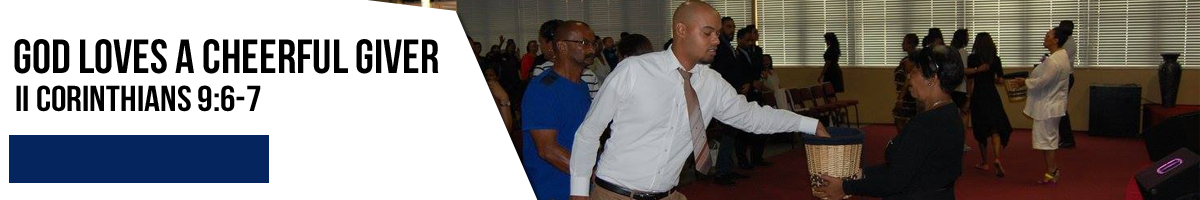# Africa ImpactPlease enter your amount in Rands. If your amount is over R1,000 please enter without the comma – Here is an example: 1800.00

Thank you for considering partnering with us to make a difference

``` function click_aa8269c461b55ad8ce1f360834f9c636( aform_reference ) { var aform = aform_reference; aform['amount'].value = Math.round( aform['amount'].value*Math.pow( 10,2 ) )/Math.pow( 10,2 ); aform['custom_amount'].value = aform['custom_amount'].value.replace( /^s+|s+\$/g,"" ); if( !aform['custom_amount'].value || 0 === aform['custom_amount'].value.length || /^s*\$/.test( aform['custom_amount'].value ) ) { alert ( 'An amount is required' ); return false; } aform['amount'].value = Math.round( aform['custom_amount'].value*Math.pow( 10,2 ) )/Math.pow( 10,2 ); } ```
 *Enter amount in rands